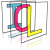Image Component Library (ICL)
icl::math::RansacFitter< DataPoint, Model > Class Template Reference

Generic RANSAC (RAndom SAmpling Consensus) Implementation. More...

`#include <RansacFitter.h>`

## Classes

struct  Result
result structure More...

## Public Types

typedef std::vector< DataPoint > DataSet
DataSet type (just a set of DataPoint instances) More...

typedef utils::Function< Model, const DataSet & > ModelFitting
Function for the fitting module (gets a dataset and returns the fitted model) More...

typedef utils::Function< icl64f, const Model &, const DataPoint & > PointError
Error function for single points. More...

## Public Member Functions

RansacFitter ()
empty constructor (creates a dummy instance) More...

RansacFitter (int minPointsForModel, int iterations, ModelFitting fitting, PointError err, icl64f maxModelDistance, int minClosePointsForGoodModel, icl64f minErrorExit=0)
constructor with given parameters More...

const Resultfit (const DataSet &allPoints)
fitting function (actual RANSAC algorithm) More...

## Private Member Functions

void find_random_consensus_set (DataSet &currConsensusSet, const DataSet &allPoints, std::vector< int > &usedIndices)
internal utility method More...

## Static Private Member Functions

static bool find_in (const std::vector< int > &v, int i, int n)
internal utility method More...

## Private Attributes

int m_minPointsForModel
minimum points that are used to create a coarse model More...

int m_iterations
number of iterations More...

icl64f m_maxModelDistance
maximum distance of a point to the model to become an inlier More...

int m_minClosePointsForGoodModel
minimum amount of inliers for a 'good' model More...

ModelFitting m_fitting
fitting function More...

PointError m_err
point-model error function More...

icl64f m_minErrorExit
min error criterion for early exit More...

Result m_result
internal result buffer More...

## Detailed Description

### template<class DataPoint = std::vector<float>, class Model = std::vector<float>> class icl::math::RansacFitter< DataPoint, Model >

Generic RANSAC (RAndom SAmpling Consensus) Implementation.

The RansacFitter provides a generic framework, for RANSAC based model fitting.

# Algorithms

The RANSAC Algorithm is well described on Wikipedia

http://de.wikipedia.org/wiki/RANSAC-Algorithmus

# Example (Image Convolution)

An example is given in the ICL Manual

# Template Parameters

The two tempalte parameters are kept very general. Therefore, there are just a few restrictions for the DataPoint and Model classes.

• default constructable
• copyable

## ◆ DataSet

template<class DataPoint = std::vector<float>, class Model = std::vector<float>>
 typedef std::vector icl::math::RansacFitter< DataPoint, Model >::DataSet

DataSet type (just a set of DataPoint instances)

## ◆ ModelFitting

template<class DataPoint = std::vector<float>, class Model = std::vector<float>>
 typedef utils::Function icl::math::RansacFitter< DataPoint, Model >::ModelFitting

Function for the fitting module (gets a dataset and returns the fitted model)

## ◆ PointError

template<class DataPoint = std::vector<float>, class Model = std::vector<float>>
 typedef utils::Function icl::math::RansacFitter< DataPoint, Model >::PointError

Error function for single points.

## ◆ RansacFitter() [1/2]

template<class DataPoint = std::vector<float>, class Model = std::vector<float>>
 icl::math::RansacFitter< DataPoint, Model >::RansacFitter ( )
inline

empty constructor (creates a dummy instance)

## ◆ RansacFitter() [2/2]

template<class DataPoint = std::vector<float>, class Model = std::vector<float>>
 icl::math::RansacFitter< DataPoint, Model >::RansacFitter ( int minPointsForModel, int iterations, ModelFitting fitting, PointError err, icl64f maxModelDistance, int minClosePointsForGoodModel, icl64f minErrorExit = `0` )
inline

constructor with given parameters

The parameters functionality is documented with the analogously named member variables

## ◆ find_in()

template<class DataPoint = std::vector<float>, class Model = std::vector<float>>
 static bool icl::math::RansacFitter< DataPoint, Model >::find_in ( const std::vector< int > & v, int i, int n )
inlinestaticprivate

internal utility method

## ◆ find_random_consensus_set()

template<class DataPoint = std::vector<float>, class Model = std::vector<float>>
 void icl::math::RansacFitter< DataPoint, Model >::find_random_consensus_set ( DataSet & currConsensusSet, const DataSet & allPoints, std::vector< int > & usedIndices )
inlineprivate

internal utility method

## ◆ fit()

template<class DataPoint = std::vector<float>, class Model = std::vector<float>>
 const Result& icl::math::RansacFitter< DataPoint, Model >::fit ( const DataSet & allPoints )
inline

fitting function (actual RANSAC algorithm)

## ◆ m_err

template<class DataPoint = std::vector<float>, class Model = std::vector<float>>
 PointError icl::math::RansacFitter< DataPoint, Model >::m_err
private

point-model error function

## ◆ m_fitting

template<class DataPoint = std::vector<float>, class Model = std::vector<float>>
 ModelFitting icl::math::RansacFitter< DataPoint, Model >::m_fitting
private

fitting function

## ◆ m_iterations

template<class DataPoint = std::vector<float>, class Model = std::vector<float>>
 int icl::math::RansacFitter< DataPoint, Model >::m_iterations
private

number of iterations

## ◆ m_maxModelDistance

template<class DataPoint = std::vector<float>, class Model = std::vector<float>>
 icl64f icl::math::RansacFitter< DataPoint, Model >::m_maxModelDistance
private

maximum distance of a point to the model to become an inlier

## ◆ m_minClosePointsForGoodModel

template<class DataPoint = std::vector<float>, class Model = std::vector<float>>
 int icl::math::RansacFitter< DataPoint, Model >::m_minClosePointsForGoodModel
private

minimum amount of inliers for a 'good' model

## ◆ m_minErrorExit

template<class DataPoint = std::vector<float>, class Model = std::vector<float>>
 icl64f icl::math::RansacFitter< DataPoint, Model >::m_minErrorExit
private

min error criterion for early exit

## ◆ m_minPointsForModel

template<class DataPoint = std::vector<float>, class Model = std::vector<float>>
 int icl::math::RansacFitter< DataPoint, Model >::m_minPointsForModel
private

minimum points that are used to create a coarse model

## ◆ m_result

template<class DataPoint = std::vector<float>, class Model = std::vector<float>>
 Result icl::math::RansacFitter< DataPoint, Model >::m_result
private

internal result buffer

The documentation for this class was generated from the following file: